TEL : +81 48 254 1744

"Easy" Gear Selection Screen

- Choose a gear by pitch, # of teeth, or bore size.
- 2D/3D Drawings Available
- PDF Catalog Available
- Strength Calculation Available

Go to Gear Selection Screen

CLOSE

# Determining the Specifications of Gears

## 16.1 Method for Determining the Specifications of a Spur Gear

Illustrated below are procedural steps to determine specifications of a spur gear.

Steps

1. Count how many teeth a sample spur gear has z =
2. Measure its tip diameter da =
3. Estimate an approximation of its module, assuming that it has an unshifted standard full depth tooth, using the equation: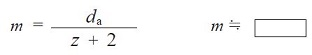4. Measure the span measurement of k and the span number of teeth. Also, measure the k –1. Then calculate the difference.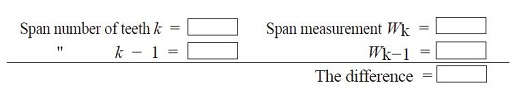5. This difference represents pb = πm cos α
Select module m and pressure angle α from the table on the right.
m =
α =
6. Calculate the profile shift coefficient x based on the above m and pressure angle α and span measurement W.
x =
To find the calculation method, please see table 5.10 (No.2) on Page 542.

Table 16.1 Base Pitch pb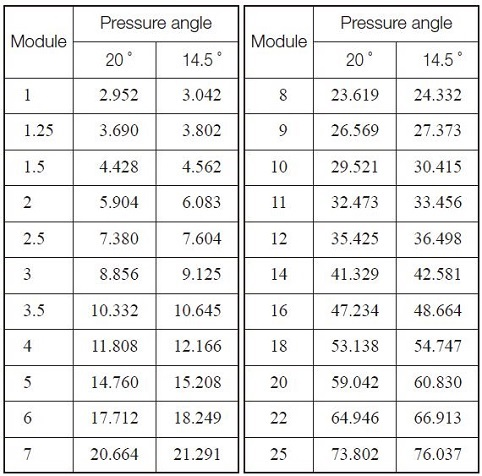NOTE.
This table deals with the pressure angle 20 deg and 14.5 deg only. There maybe cases where the degree of the pressure angle is different. Tooth profiles come with deep tooth depth or shallow tooth depth, other than a full tooth depth.

## 16.2 Method for Determining the Specifications of a Helical Gear

The Helix angle is what differs helical gears from spur gears and it is necessary that the helix angle is measured accurately. A gear measuring machine can serve this purpose, however, when the machine is unavailable you can use a protractor to obtain an approximation.
Lead pz of a helical gear can be presented with the equation: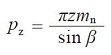Given the lead pz, number of teeth z normal module mn, the helix angle β can be found with the equation: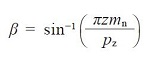The number of teeth z and normal module mn can be obtained using the method for spur gears explained above. In order to obtain pz, determine da by measuring the tip diameter. Then by using a piece of paper and with ink on the outside edge of a helical gear, roll it on the paper pressing it tightly. With a protractor measure the angle of the mark left printed on the paper, βa. Lead pz can be obtained with the following equation.

Fig. 16.1 The measurement of a helix angle on tooth tips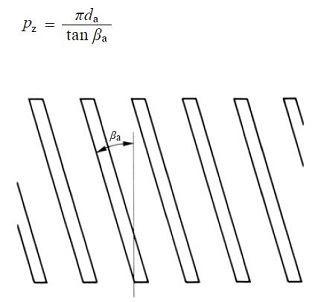Go to Top